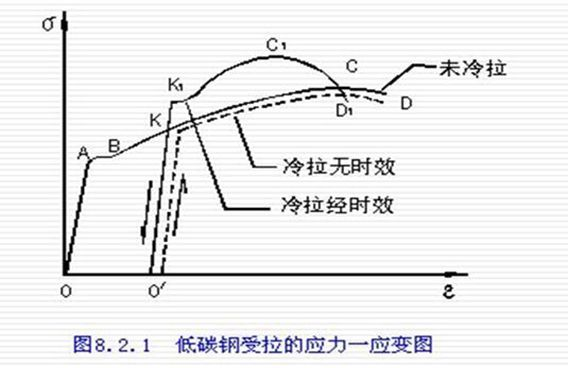# “弹性模量”的值是越大越好还是越小越好？

• A+

### 问题内容

“弹性模量”的值是越大越好还是越小越好？ 视情况而定，弹性模量越低，弹性模量变形相对越大，刚度越小，材料易发生变形柔性越好；弹性模量越高，材料发生弹性模量变形相对越小，刚度大，材料不易变形，脆性越强。

### 网友回答1兆帕(MPa)=145磅/英寸2(psi)=10.2千克力/平方厘米(kgf/cm²)=10巴(bar)=9.8大气压(atm)

1磅/英寸2(psi)=0.006895兆帕(MPa)=0.0703千克力/平方厘米(kgf/cm²)=0.0689巴(bar)=0.068大气压(atm)

1巴(bar)=0.1兆帕(MPa)=14.503磅/英寸2(psi)=1.0197千克力/平方厘米(kgf/cm²)=0.987大气压(atm)

1大气压(atm)=0.101325兆帕(MPa)=14.696磅/英寸2(psi)=1.0333千克力/平方厘米kgf/cm²)=1.0133巴(bar)